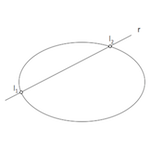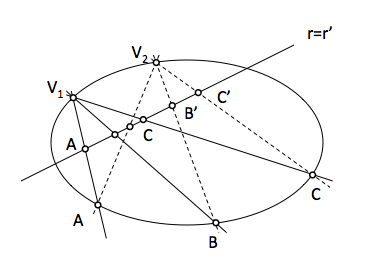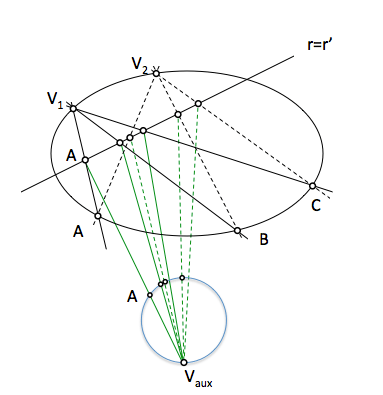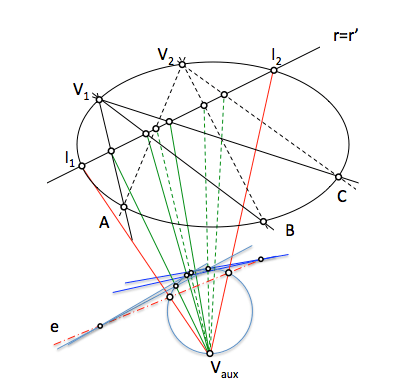# Geometría proyectiva: Intersection of straight and taperedThe defining the conical projective lets start solving classic problems of identification of new elements of the conical (new points and tangents in them), and find the intersection with a line or a tangent from an external point. These problems can be solved by various more or less complex methods and conceptually more or less laborious paths.

We will now see how to determine the two possible intersection points of a line with a taper defined by five points. As an auxiliary tool to use a resolution circumference as a series of second order.The problem is determined by 5 points (P1 … P5) and a line r. The conical not find mapped. The analysis is shown to serve as conceptual support but we can not use the curve directly in solving the problem.By understanding the conical as a curve contains points produced by the intersection of two projective beams and further containing at the vertices of said beams, we can generate two beams with two vertex points and project the remaining three of the conical rays to find their.

It section the line r by the rays of these projective bundles obtain two overlapping series of first-order the base line in which we determine the points of intersection.The points of intersection we seek will be double series of overlapping elements, so the problem is reduced to obtain two superimposed double series elements.

To resolve this problem will project from an auxiliary point (Vaux) to pass to the overlapping concentric series and then do seccionaremos a circle through the new vertex. This series will get second order on the circumference which are projective series of overlapping on the line.To determine the dual elements in the series will get its second order projective axis, double points being the cut of this axis with the circular base. In case of a single two-point tangent to the line would be the conic, and if there were any (projective shaft outer circumference) r not cut straight to the conical.